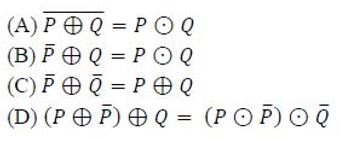Related Articles
GATE | GATE CS 2018 | Question 21
• Last Updated : 20 Nov, 2018

Let ⊕ and ⊙ denote the Exclusive OR and Exclusive NOR operations, respectively. Which one of the following is NOT CORRECT?(A) A
(B) B
(C) C
(D) D

Answer: (D)

Explanation: (A) (p⊕q)’ = (pq’ + p’q)’ = (p’+q).(p+q’) = (pp’ +p’q’ + qp + qq’) = pq + p’q’ = (p⊙q)
(B) (p’)⊕q = (p’)q’ + (p’)’q = pq + p’q’ = (p⊙q)
(C) (p’⊕q’) = (p’)’.(q’) + (p’).(q’)’ = p.(q’) + (p’).q = p⊕q.
(D) (p⊕(p’))⊕q =(pp”+ p’p’)⊕q =(p+p’)⊕q = 1⊕q = 1.q’ + 0.q = q’
And
(p⊙(p’))⊙(q’) = (pp’ + p’.p”)⊙(q’) = 0⊙(q’) = 0(q’) + 1.(q’)’ = q

Please note that (p⊕q) = (p’⊙q) = (p⊙q’) = (p’⊙q’)’ but not (p’⊙q’).
Similarly, (p⊙q) = (p’⊕q) = (p⊕q’) = (p’⊕q’)’ but not (p’⊕q’).

So, option (D) is false.

Quiz of this QuestionMy Personal Notes arrow_drop_up